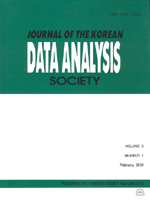상세검색
최근 검색어 전체 삭제
다국어입력
즐겨찾기0KCI등재 학술저널

# Nonparametric Survival Analysis in Time-Varying Coefficient Models

• 등재여부 : KCI등재
• 2011.06
• 1101 - 1109 (9 pages)

The Cox model has been widely used for the analysis of survival data under the proportional hazards assumption. The subject of examining the hazard proportionality assumption in survival data analysis has been studied extensively. One of methods for modeling nonproportional hazards is to use time-varying coefficient functions associated with covariates. In this paper, we propose a simple nonparametric method for estimating timevarying coefficient functions in hazard regression model via penalized likelihood by using a lower-dimensional approximation. Also, Bayesian confidence intervals are computed derived from Bayes model for the lower-dimensional approximations. The proposed method is illustrated by using a real data on patients diagnosed with AIDS in Australia.

1. Introduction

2. Penalized Likelihood Estimation

3. Computation

4. Data Analysis

5. Conclusion

References